# Sketch the V-I characteristics of triac . Describe some of its important applications

## V-I characteristics of triac

In this article we will sketch  and explain the V-I characteristics of triac and also discuss some of its important applications.

Fig.1 shows the V-I characteristics of triac .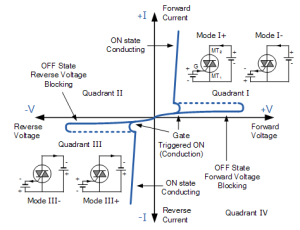Fig.1

Because the triac essentially consists of two SCRs of opposite orientation fabricated in the same crystal, its operating characteristics in the first and third quadrants are the same except for the direction of applied voltage and current flow.

The following points may be noted from the triac characteristics:

1. The V-I characteristics for triac in the Ist and IIIrd quadrants are essentially identical to those of an SCR in the Ist quadrant.
2. The triac can be operated with either positive or negative gate control voltage but in normal operation usually the gate voltage is positive in Ist quadrant and negative in IIIrd quadrant.
3. The supply voltage at which the triac is turned ON depends upon the gate current. The greater the gate current, the smaller the supply voltage at which the triac is turned on. This permits to use a triac to control a.c. power in a load from zero to full power in a smooth and continuous manner with no loss in the controlling device.

### Triac Phase Control Circuit

A triac can be used to control the average a.c. power to a load by passing a portion of positive and negative half cycles of input a.c.

This is achieved by changing the conduction angle through the load.

Fig.2 shows the basic triac phase control circuit.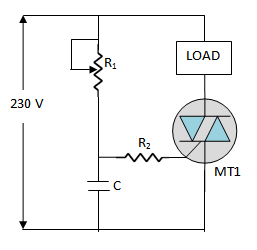Fig.2

This circuit uses a capacitor C and variable resistance R1 to shift the phase angle of the gate signal.

Because of this phase shift , the gate voltage lags the line voltage by an angle between 0o and 90o .

By adjusting the variable resistance R1, the conduction angle through the load can be changed.

Thus any portion of positive and negative half-cycles of the a.c. can be passed through the load.

This actin of triac permits it to be used as a controlled bidirectional switch.

#### Circuit Action

The operation of triac phase control circuit is as under:

(i) During each positive half-cycle of the a.c.,the triac is off for a certain interval, called firing angle α (measured in degrees) and then it is triggered on and conducts current through the load for the remaining portion of the positive half-cycle, called the conduction angle ΘC.

The value of firing angle α and hence ΘC can be changed by adjusting the variable resistance R1.

If Ris increased, the capacitor will charge more slowly, resulting in the triac being triggered later in the cycle i.e. firing angle α is increased while conduction angle ΘC is decreased.

As a result, smaller a.c. power is fed to the load.

Reverse happens if the resistance R1 is decreased.

(ii) During each negative half-cycle of the a.c., a similar action occurs except that now current in the load is in the opposite direction.

Fig.3 shows the waveforms of the line voltage and gate voltage.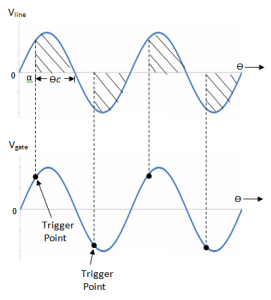Fig.3

Only the shaded portion of the positive and negative half-cycles pass through the load.

We can change the phase angle of gate voltage by adjusting the variable resistance R1 .

Thus a triac can control the a.c. power fed to a load. This control of a.c. power is useful in many applications such as industrial heating, lighting etc.

### Applications of Triac

As low gate current and voltages can be used to control large load currents and voltages, therefore, triac is often used as an electronic on/off switch controlled by a low-current mechanical switch.

#### (i) As a High-Power Lamp Switch

Fig.4 shows the use of a triac as an a.c. on/off switch.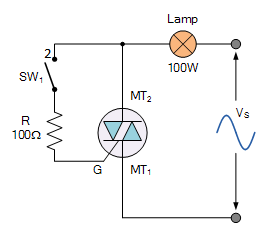Fig.4

When switch SW is thrown to position 1, the triac is cut off and the output power of lamp is zero.

But as the switch is thrown to position 2, a small gate current (a few mA) flowing through the gate turns the triac on.

Consequently, the lamp is switched on to give full output of 100 watts.

#### (ii) Electronic change over of transformer taps

Fig.5 shows the circuit of electronic change over of power transformer input taps.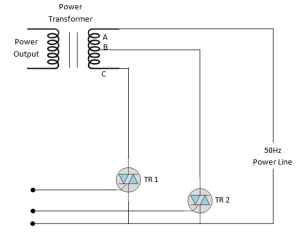Fig.5

Two triacs TR1 and TR2 are used for the purpose.

When triac TR1 is turned on and triac TR2 is turned off, the line input is  connected across the full transformer primary AC.

However, if it is desired to change the tapping so that input appears across part AB of the primary, then TR2 is turned on and TR1 is turned off.

The gate control signals are so controlled that both triacs are never switched on together.

This avoids a dangerous short circuit on the section BC of the primary.